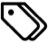writing | programming | development | octopress | website

I’ve been meaning to try and implement MathJax on this website for a while now. For including math equations on a website, MathJax is probably one of the more elegant ways to do it. I can write equations in TeX format, and MathJax renders the equations properly for you!

Finally, in the last couple of days I’ve been forced to get around to it, thanks to a new post that I’m writing that includes a little bit of math. So anyway, I just wanted to jot down that process.

The thing with MathJax is that it’s meant to, and does, work with HTML. But since I’m working with Octopress and Markdown, I have to ensure that the conversion from Markdown to HTML produces no unwanted syntactical errors for MathJax. To get around this problem, Zac Harmany (I hope I got the name right) suggests tweaking the Markdown rendering engine to Pandoc, so that the conversion works as desired. I’m sure that works great, but I had no intention of tweaking my Markdown conversion engine. Instead, I discovered a nifty ruby bundle (here) that serves a great purpose.

Next, the typical MathJax “installation” involves adding a line in the <head> section of your Octopress theme (Add it to %octopress_root%/source/_includes/custom/head.html), so that when every page loads, the necessary Javascript files are also loaded, ready to render your equations. I did not want the Javascript to execute to load along with all my pages, given that I don’t expect to have equations in all my posts. Instead, I’ve included the call to the script in the <body> of the post, i.e. in the meat of the post itself. Remember that the declaration needs to be before your first equation.

I also contemplated downloading the MathJax distribution and hosting it locally on my own server, but that did not work at all. There are way too many files to be uploaded (each file is small; the total package is ~50MB; there are too many files, though) and it just took forever to upload to my server until I just gave up. I’ll revisit that option if I think using MathJax’s own servers is not working well—which I doubt will happen.

With those details, here’s how I set things up:

• Install the verbatim.rb plugin from this Github repository. To do this, simply download the file (‘Gist’ in Github parlance) and place it in %octopress_root%/plugins. When inserting equations, there’s a syntax to using this plugin; I’ll demo it below.

• Create a MathJaxLocal.js file at %octopress_root%/source/javascripts/ to add local configurations for MathJax. Note that the last line of the code must point to the full path of the local file, in my case http://arnabocean/javascripts/MathJaxLocal.js

Here’s what my MathJaxLocal.js looks like (I started with Zac Harmany’s file and modified to suit my needs.):

MathJax.Hub.Config({
jax: ["input/TeX","output/HTML-CSS"],
tex2jax:
{
inlineMath: [ ['$','$'], ['\$$','\$$'] ],
displayMath: [ ['$$','$$'], ['\$','\$'] ],
skipTags: ["script","noscript","style","textarea","pre","code"],
processEscapes: true
},
TeX:
{
equationNumbers: { autoNumber: "AMS" },
TagSide: "left",

},
"HTML-CSS": { availableFonts: ["TeX"] }
});

• Declare the location of the MathJax files. The easiest thing to do is to use MathJax’s own servers. However, in addition to just their servers, you’ll have to link to your own local config file as well, so we’ll add both of these at the same time. In the main body of your markdown post (preferably after the “Read More” fold), add the following:

<script type="text/javascript"
src="http://cdn.mathjax.org/mathjax/latest/MathJax.js?config=TeX-AMS-MML_HTMLorMML,http://arnabocean.com/javascripts/MathJaxLocal.js">
</script>


In the above, the first link points to MathJax servers, the second points to my own config file.

And that’s basically it! Now you’re all set to write beautiful equations. Here’s a demo:

<!-- MathJax configuration -->
<script type="text/javascript" src="http://cdn.mathjax.org/mathjax/latest/MathJax.js?config=TeX-AMS-MML_HTMLorMML,http://arnabocean.com/javascripts/MathJaxLocal.js">
</script>
<!-- End MathJax Configuration -->

{% raw %}{% verbatim tag:p %}{% endraw %}
$f(x)= a_0 + a_1\sin(x) + a_2\sin(2x) + ...$

$+b_1\cos(x) + b_2\cos(2x) + ...$

$f(x)=a_0+\sum_{k=1}^\infty\big( a_k\cos(kx)+b_k\sin(kx) \big)$
{% raw %}{% endverbatim %}{% endraw %}


And here’s what that would look like:

$f(x)= a_0 + a_1\sin(x) + a_2\sin(2x) + …$ $+b_1\cos(x) + b_2\cos(2x) + …$ $f(x)=a_0+\sum_{k=1}^\infty\big( a_k\cos(kx)+b_k\sin(kx) \big)$

There’s still a lot of more that I need to find out, and from the looks of it, Zac’s website is a great resource. I’ll add more posts if I find anything useful that I end up using.